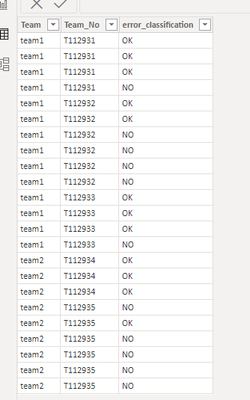cancel
Showing results for
Did you mean:Frequent Visitor

## Average of pbix and excel pivot table not matching

Hi all,

Hope someone can help me out with my question.

The average I get when calculating with dax is different then calculation in excel pivot table (see picture below).

I understand how the calculation is different, but I can't get it work, to get both calculations the same.

Can anybody help me out ?

Regards,

Yvonne1 ACCEPTED SOLUTIONCommunity Support

Hi , @Yvonne24

According to your description, you want to change the calculation on the %OK in Matrix.

Here are the steps you can refer to :
(1)This is  my test data:(2)We can create four measures to compare:

#total = COUNT('Table'[error_classification])

#OK = CALCULATE( COUNT('Table'[error_classification]), FILTER('Table','Table'[error_classification]="OK"))

% OK in Power BI = [#OK] / [#total]

% OK in Excel = var _flag =  NOT( ISINSCOPE('Table'[Team_No]))
var _average = AVERAGEX( ADDCOLUMNS(VALUES('Table'[Team_No]),"percentage" , [% OK in Power BI]) , [percentage])
return
IF(_flag= TRUE() , _average , [% OK in Power BI])

(3)Then we can out these measures on the visual and we can meet your need:If this method does not meet your needs, you can provide us with your special sample data and the desired output sample data in the form of tables, so that we can better help you solve the problem.

Thank you for your time and sharing, and thank you for your support and understanding of PowerBI!

Best Regards,

Aniya Zhang

If this post helps, then please consider Accept it as the solution to help the other members find it more quickly

4 REPLIES 4Community Support

Hi , @Yvonne24

According to your description, you want to change the calculation on the %OK in Matrix.

Here are the steps you can refer to :
(1)This is  my test data:(2)We can create four measures to compare:

#total = COUNT('Table'[error_classification])

#OK = CALCULATE( COUNT('Table'[error_classification]), FILTER('Table','Table'[error_classification]="OK"))

% OK in Power BI = [#OK] / [#total]

% OK in Excel = var _flag =  NOT( ISINSCOPE('Table'[Team_No]))
var _average = AVERAGEX( ADDCOLUMNS(VALUES('Table'[Team_No]),"percentage" , [% OK in Power BI]) , [percentage])
return
IF(_flag= TRUE() , _average , [% OK in Power BI])

(3)Then we can out these measures on the visual and we can meet your need:If this method does not meet your needs, you can provide us with your special sample data and the desired output sample data in the form of tables, so that we can better help you solve the problem.

Thank you for your time and sharing, and thank you for your support and understanding of PowerBI!

Best Regards,

Aniya Zhang

If this post helps, then please consider Accept it as the solution to help the other members find it more quicklyFrequent Visitor

Wow this is amazing!

Been working on this for a long time.

Thank you very much!!!!

YvonneSolution Sage

The difference in the average calculation between Power BI and Excel could be due to several factors. Here are a few possibilities:

1. The data source: Are you using the same data source in both Power BI and Excel? If not, there may be differences in the data that are affecting the average calculation.

2. The calculation method: Power BI uses the DAX language to calculate measures, while Excel uses its own calculation engine. There may be differences in how the averages are calculated.

3. Filtering: Are you applying any filters in Power BI that are not being applied in Excel? This can affect the average calculation.

4. The data type of the field: Make sure that the data type of the field is the same in both Power BI and Excel. Also, check that the field is not formatted in a way that could affect the average calculation.

5. Missing values: If there are missing values in the dataset, the way how it is handled can cause a difference in the average calculation.

In order to resolve this issue, you can try to use the same data source and calculation method in both Power BI and Excel and ensure that the data type of the field and filtering are the same. Also, you can check for missing values and if exist try to filter them out or if not possible to handle them in a way that works for your analysis.Frequent Visitor

I use the same data as I extract the data from the PowerBi table (report) into excel. So therefor the same filtering is present and no missing values. The calculation methode is indeed different. But I do not know how to create a DAX that gives the same result as the pivot table (or visa versa).